﻿ Analysis on Hawking Radiation and Steady State Universe by Siva’s TheoriesOPEN ACCESSPEER-REVIEWED

Siva Prasad Kodukula1,2,1Adviser- Centre for Innovation & Discovery, Bheemunipatnam-, India
2Employee of Jindal Steel & Power Ltd, Angul, India

Abstract

Every black hole will have a singularity at its center. The mass ‘M’ and Horizon radius ‘R’ are inter related by an equation M3 = 2.914× 1038 R. As long as it remains as a stable black hole the mass ‘M’ & Horizon Radius ‘R’ will remain constant with M = 6.58×105 Kgs and R = 9.767×10-22 mts. This is the hole connecting all the singularities of the universe. These are always constant for all black holes of the universe. Heavy black holes will lose mass through this hole and will be pumped to other locations of universe to keep the density of the matter created in steady state with the expansion of the universe. The mass loss calculated is 4.953083423× 105 Kg/sec. The mass loss is almost same as that of life time of black hole in Hawking Radiation. Thus the mass loss of black hole is not through hawking radiation it is through the singularity point of black hole.

At a glance: Figures

1
Prev Next

• Kodukula, Siva Prasad. "Analysis on Hawking Radiation and Steady State Universe by Siva’s Theories." International Journal of Physics 2.2 (2014): 30-33.
• Kodukula, S. P. (2014). Analysis on Hawking Radiation and Steady State Universe by Siva’s Theories. International Journal of Physics, 2(2), 30-33.
• Kodukula, Siva Prasad. "Analysis on Hawking Radiation and Steady State Universe by Siva’s Theories." International Journal of Physics 2, no. 2 (2014): 30-33.

 Import into BibTeX Import into EndNote Import into RefMan Import into RefWorks

1. Introduction

‘Siva’s Classical Equations for Space Time and Matter’  describes how to calculate the space time fluid density for any mass. The equations are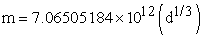(1)(2)

Where ‘γ’ is ‘space time fluid density’ of mass ‘m’

The same concept has been applied to massive black holes. The complete black hole is a mass spread over a radius and a singularity  at its center. ‘Virtual K-Suryon’[9, 11] is a singularity with zero mass. But black hole is a singularity with mass. The difference is space time.

K-Suryon will not have space time. But black hole will have space time and a singularity at its center. It follows equation for singularity and Schwarzschild radius.

2. DiscussionSo we can find the ‘R’ for a black hole of mass MB, It will increase as the mass increases.

This shows the increment in surface area of Horizon for a black hole with mass. Let us suppose a mass from outside of black hole has been swallowed by a black hole of mass ‘MB’ so that the mass of black hole has become ‘n’ times i.e ‘nMB ’.

For this ‘nMB we can calculate the space time fluid density.

As per equation (1) the radius of space time fluid can be calculated.

If we substitute the ‘nMB’ in place of ‘m’ of equation (1)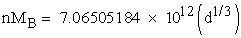(3)

where ‘d’ is space time fluid radius of newly formed black hole.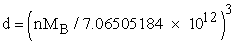(4)

If we substitute this value of ‘d’ in equation (2)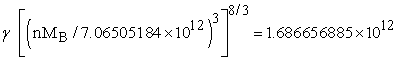Therefore(5)

Density of new black hole can be calculated as-Radius is ‘R’ and volume = (4/3)π R3(6)

Equation (5) can be equated with equation (6)

ThereforeTherefore(7)

We can say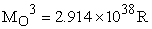(8)

where ‘MO’ is mass of newly formed black hole with radius ‘R’.

We have the relation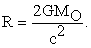We have G = 6.67×10-11, C= 2.997925×108 mt/sec.

Therefore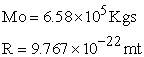Thus we can say that the mass ‘M’ and Horizon radius ‘R’ of a black hole is inter related by an equation M3 = 2.914× 1038 R. Thus all the stable black holes with mass ‘M’ & Horizon Radius ‘R’ will remain constant with M = 6.58×105 Kgs and R = 9.767×10-22 mts. This is the hole connecting all the singularities of the universe. These are always constant for all black holes of the universe.

If this is the case, when a star becomes a black hole, the mass and the Schwarzschild radius of that black hole will be higher than the above calculated constant parameters i.e M = 6.58×105 Kgs and R = 9.767×10-22 mts. So it is understood that the black hole will not be stable and it will loose its mass through the hole surrounded by its singularity point existed at its center. This is the hole connecting all the singularities of the universe.

This mass will be redistributed to the universe since it requires continuous creation of matter to maintain in steady state with expansion.

As per the “Heart of God Model’ of the universe, the universe is like human heart pumps the matter in to this universe for every 7.6813002x10-44 seconds which is the duration of film change of the universe as per ‘Film theory of the Universe’ [5, 6]. Figure 1 shows the graphical representation of films in this universe. The pumping of new matter is a continuous process in this universe.. The density of the matter will be constant. The creation of matter will be compensated by expansion so as to maintain the density of matter of the universe even though it is expanding. This is similar result of Sir Fred Hoyle’s steady state theory.

Thus the density of Mass draining per second through singularity point of black hole = the density of the mass pumped out of ‘Heart of God’ per second.

2.1. Calculation for Loss of Mass of Black Hole

Calculation for the loss of density of mass for the black hole per second through its own Singularity:

We have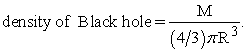And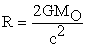(9)

This singularity will be connected to all the singularities of the universe including ‘K-Suryon’, the basic building block of matter.

As per the concept, the mass lost through its singularity = the mass pumped out by ‘Heart of the God model’.

Already ‘Heart of God model’ Explained and calculated the density of the mass creation in one second.

2.2. Review of the Calculation by ‘Heart of God Model’

According to film theory, universe is made up of films which are changing for every 7.6813002x10-44 sec. The events are occurring due to change of film. It is like a movie in which sixteen films are changing at every second. In ‘Double Relativity’ it is described that a force will act according to equation V=Hd in one direction and Vd=K in opposite direction. Even though time is a quantized quantity the space-time is not. The space-time is a continuous one. So how to make this in to one system which obeys continuous expansion of space with time. So there must exist something in our conventional four-dimensional world, which we have described as nothing in full truth. Already we have described that light is that nothing which seems like photon in this four-dimensional space-time. The same thing will exist between two films. This is a singularity concept of ‘K-suryon’ explains the creation of mass from this singularity. So the process of continuous creation of matter exists in between two films of the universe.

2.3. Calculation for Quantity of Mater Creates during Film Change 

Let us suppose, for the first film, space of the universe is with diameter ‘do’ and the matter is existed with velocity ‘Vo’. It can be related with the equation Vo=H do. As per Double relativity Effect the Vmin =1000mts/sec. Since it is first film.

Therefore it’s diameter ‘do’ = Vmin / H = 1.9147644×1022 mts. Where ‘H’ is Hubble’s constant i.e equal to 5.223×10-20 /sec as per ‘derivation of Siva’s Constant ‘K’.

2.3.1. Data For the First Film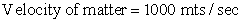Time taken for film change i.e ‘t’ =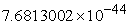sec.

So with in this time the matter at a point at ‘do’ dia will move to a dia of ‘d’ i.e. dia of second film.

So we can calculate change of dia during film change.

2.3.2. Data for the Second Film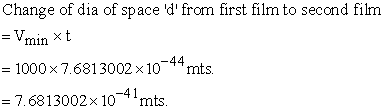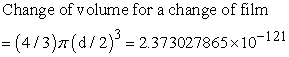Let us keep the density of matter constant and compensate the expansion.

For(As calculated in ‘Double Relativity Effect’) .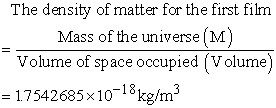For second film,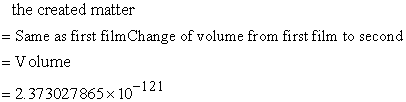(As per above calculation)Duration of film change [5, 6] ‘t’ =secThis is constant. It will not change with film change. For first film, second film it is same

Therefore finally the density of the matter created while film change, can be calculated by distributing the created matter throughout its constant volumeThis is similar result as predicted by ‘steady state theory’ which states that universe is always existed in a steady state, the expansion of the universe is compensated by the continuous creation of matter which is viewed as a property of space, and that despite local evolutionary process, the universe as a whole is not evolving. The rate at which matter would have to be spontaneously created to compensate for the universe’s expansion (about 10-43 g cm-3 sec-1) .

As calculated above the density of mass Created during film change of the universe = 2.28381713×1025 Kg/m3

As per ‘Film Theory of the Universe’  no. of Films per second =1/7.6813002×10-44.

Therefore total density of matter created or pumped outThis is quantitatively equal to equation (9)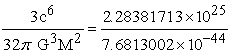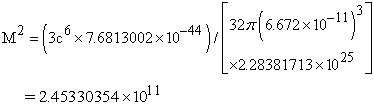∴ M = 4.953083423×105 Kg.

So we can conclude the mass loss (M) of black hole per second = 4.953083423×105 Kg.

Thus we can conclude that the mass of a black hole will be drained out through a hole which connects all the singularities or the points from the matter pumped out by ‘Heart of the God’.

The density of the matter drained out per second is equal to the density of matter created per second.

Thus we reached a conclusion that the mass of black hole will be drained out through its singularity as a mass loss.

Similar concept of mass loss has been calculated by black hole physics in terms of ‘Hawking Radiation’ .

2.3.3. Hawking Radiation in Terms of Mass Loss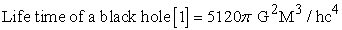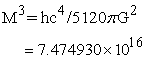Mass loss per second = 4.212459223×105 Kg.

This is almost equal to the mass loss of black hole to pump out the matter by ‘Heart of God’ to compensate expansion of the universe as well as to keep the universe in steady state i.e. 4.953083423×105 Kg.

•  This analysis elaborates the process of matter creation as explained in steady state theory or ‘Heart of the God model’ to compensate the expansion of universe.

•  The matter absorbed by the black holes will be spread over the universe in some other area.

•  The concept of ‘Film theory of Universe’ ‘Heart of the God model’ is in line with the present day calculations of black hole physics and black hole thermodynamics.

•  The calculations and the idea of mass loss of black hole are correct but not as expected as Hawking Radiation. The mass loss is through the hole surrounded by singularity existed at its center and further will be divided in to K-Suryons to pump out as a continuous creation of matter to keep the universe in steady state.

•  The rate of mass loss of black holes i.e 4.953083423× 105 Kg/sec can be observed by ‘molecular gas kinematics’.

3. Conclusions

1. The mass ‘M’ and Horizon radius ‘R’ are inter related by an equation M3 = 2.914×1038 R.

2. The mass ‘M’ and Horizon radius ‘R’ of all black holes are same M = 6.58×105 Kgs and R = 9.767×10-22 mts.

3. Black hole with above parameters is the hole connecting all the singularities of the universe.

4. All the black holes which are greater in mass will loose its mass through its singularity point at a rate 4.953083423× 105 Kg /sec.

5. The mass lost through this singularity point will compensate the creation of rate of density of matter pumped out by ‘Heart of the God ’ so that the expansion of the universe compensate with creation of matter. And the mass loss is almost equal to the mass loss of black hole calculated by life time calculation of ‘Hawking Radiation’.

6. Hawking radiation expecting the mass loss from the black hole as radiation (or similar explanations like particle pair in quantum black holes [2, 3, 4]) which is not supported by the analysis.

7. The rate of mass loss from the black hole can be observed by ‘molecular gas kinematics’ .

References

  Adam R. Brown, “Tensile Strength and the Mining of Black Holes” Phys. Rev. Lett. 111, 211301 (2013). http://arxiv.org/abs/1207.3342. [Accessed March. 1, 2014].In article CrossRef  George, F R Ellis. “Astrophysical black holes may radiate but they do not evaporate.” arXiv: 1310.4771v2 [gr-qc] 20 Oct 2013 Available: http://arxiv.org/pdf/1310.4771.pdf. [Accessed March. 2, 2014].In article  Hawking,S.W., “Particle creation by Black holes”. commun. 43, 199 -220 (1975) maths.phys. Available: http://arxiv.org/pdf/hep-th/9203052.pdf. [Accessed March. 2, 2014].In article  Hawking, S.W. & Penrose. The Nature of space and Time, Princeton University press, pp. 38-60, 1998.In article  Kodukula, S.P., Double Relativity Effect &Film Theory of the Universe. Raleigh,NorthCorolina:Lulu.com, pp 13-32, 50, 2009.In article  Kodukula S.P,Heart of the God with Grand proof Equation - A classical approach to quantum theory. Raleigh, NorthCorolina:Lulu.com, pp3-9, 53, 2009.In article  Kodukula, S.P, “Derivation of Siva’s Constant of Physics”, International Journal of Advancements in Research & Technology’, 2(1), Jan. 2013. Available: http://www.ijoart.org/docs/Derivation-of-Sivas-Constant-K-of-Physics.pdf. [Accessed March. 1, 2014].In article  Kodukula, S.P, “Siva’s Classical Equation for Space Time and Matter”, International Journal of Advancements in Research & Technology’, 2(8), Aug. 2013. Available: http://www.ijoart.org/docs/Sivas-Classical-Equation-for-Space-Time-and-Matter.pdf. [Accessed March. 1, 2014].In article  Kodukula. S.P, “Siva's Theory of Quantum Gravity”. American Journal of Modern Physics, 3(1), 16-19. Jan. 2014. [Accessed March. 1, 2014].In article CrossRef  Kodukula,S.P,“Siva’s Equation for Singularity of Black Holes”. International Journal of Astrophysics and Space Science,1(4),16-19. 2013.In article  Kodukula, S.P, “New Discovery about Prediction of a Particle ‘K-Suryon’ as Basic Building Block of Mass”. International Journal of physics, 2(1), 12-14. 2013,In article  Timothy A. Davis et al., “A black-hole mass measurement from molecular gas kinematics in NGC4526” arXiv:1301.7184v1 [astro-ph.CO] 30 Jan 2013 Available: http://arxiv.org/pdf/1301.7184.pdf [Accessed March. 2, 2014].In article  H. Bondi & T. Gold, “The Steady State Theory of the Expanding Universe” Monthly Notices of Royal Astronomical Society, Vol.108, p. 265 (1948). Available: http://adsabs.harvard.edu/full/1948MNRAS.108..25B [Accessed April 5,2014].In article